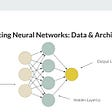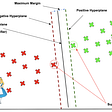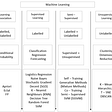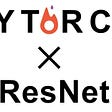# Model Deployment

Model Deployment is the method by which you integrate a machine learning model into an existing production environment to make practical business decisions based on data. It is one of the last stages in the machine learning life cycle and can be one of the most cumbersome. [Definition source]

Model deployment is probably the most important part of the Machine Learning model lifecycle but still, the least studied one. Most of the courses out there around the ML/DL universe teach how to explore data, engineer the features, train the model, and generate predictions. But they miss the most important part: what to do after that?

Apart from the models developed for learning or for Kaggle competitions, all other models are built to generate revenue, and if you don't deploy a model into production then there's no one using it and thus no revenue.

# ONNX vs Vendor lock-in

Sometimes you create models using one framework, for example, coreML but you want to deploy it into a system that is not supported by it (for example, Android). This non-interoperability means that your trained, tested model is of no use as-is.

One possible and obvious solution is to retrain your model using a format supported by Android. But is this obvious solution feasible? In a production environment where there are strict timelines, solutions such as this are non-optimal. And even if you have an ample amount of time, retraining is not the best solution. Training a model and taking it to optimum accuracy is no easy task. There are hours and hours of training and tuning involved which can be very cumbersome. So when you have a model already at its optimum accuracy you don’t want to retrain it from the scratch again. Taking a quote from software engineering:

First rule of programming: If it works don’t touch it.

One better alternative is to convert your model into a framework that is supported by the system where you want to deploy your model. And that's where ONNX comes into the picture.

ONNX stands for Open Neural Network Exchange. ONNX is an open-source artificial intelligence ecosystem that can be used for exchanging deep learning models. It promises to make deep learning models portable thus preventing vendor lock-in.

In September 2017 Facebook and Microsoft introduced it for switching between machine learning frameworks such as PyTorch and Caffe2. Later, IBM, Huawei, Intel, AMD, ARM, and Qualcomm announced support for the initiative.

Below, I will explain the process of converting a Pytorch model into a Keras model using ONNX (Similar methods can be used to convert between other types of models). We will use the following use case for the demo.

# Use Case: Handwritten Digits Classification

Use case brief: We want to create an ML model that can take an image as input and successfully classify it as a digit between 0 and 9.

we will create a Multi-Layered Perceptron (MLP) network for building a handwritten digit classifier. We will make use of the MNIST dataset included in the torchvision package.

We will use different utility packages provided within PyTorch (NN, autograd, optim, torchvision, torchtext, etc.) to build and train neural networks. For ease of usage and accessibility, we will be using Google Colab to run our code.

Lets get our hands dirty.

# Installation

Install the necessary packages which don't come with Google Colab by default. If you are running outside Google Colab then you need to install all the packages mentioned in the last part of this article.

`! pip install onnx! pip install onnx2keras! pip install onnxruntime`

The first step, as with any ML project you’ll work on, is data preprocessing. We need to transform the raw dataset into tensors and normalize them in a fixed range. The torchvision package provides a utility called transforms which can be used to combine different transformations together.

`from torchvision import transforms_tasks = transforms.Compose([transforms.ToTensor(),  transforms.Normalize((0.5,), (0.5,))])`

The first transformation converts the raw data into tensor variables and the second transformation performs normalization using the below operation:

x_normalized = x-mean / std

`from torchvision.datasets import MNIST## Load MNIST Dataset and apply transformationsmnist = MNIST("data", download=True, train=True, transform=_tasks)`

# Data Visualization

Lets now take a look at the dataset

`from torch.utils.data import DataLoaderrawData = DataLoader(mnist, batch_size=10)dataiter = iter(rawData)data, labels = dataiter.next()data = data.view(data.shape, -1)print("shape", data.shape)`

Out[ ]:

`shape torch.Size([10, 784])`

This dataset contains images of handwritten numbers. Each image (originally a matrix of shape 28×28) is squeezed into a vector of shape 1×784. We can get the original image by reshaping the vector into a 28×28 matrix.

This is how starting 200 values of 1st vector looks like:

`data[:200]`

Out[ ]:

`tensor([-1.0000, -1.0000, -1.0000, -1.0000, -1.0000, -1.0000, -1.0000, -1.0000,        -1.0000, -1.0000, -1.0000, -1.0000, -1.0000, -1.0000, -1.0000, -1.0000,        -1.0000, -1.0000, -1.0000, -1.0000, -1.0000, -1.0000, -1.0000, -1.0000,        -1.0000, -1.0000, -1.0000, -1.0000, -1.0000, -1.0000, -1.0000, -1.0000,        -1.0000, -1.0000, -1.0000, -1.0000, -1.0000, -1.0000, -1.0000, -1.0000,        -1.0000, -1.0000, -1.0000, -1.0000, -1.0000, -1.0000, -1.0000, -1.0000,        -1.0000, -1.0000, -1.0000, -1.0000, -1.0000, -1.0000, -1.0000, -1.0000,        -1.0000, -1.0000, -1.0000, -1.0000, -1.0000, -1.0000, -1.0000, -1.0000,        -1.0000, -1.0000, -1.0000, -1.0000, -1.0000, -1.0000, -1.0000, -1.0000,        -1.0000, -1.0000, -1.0000, -1.0000, -1.0000, -1.0000, -1.0000, -1.0000,        -1.0000, -1.0000, -1.0000, -1.0000, -1.0000, -1.0000, -1.0000, -1.0000,        -1.0000, -1.0000, -1.0000, -1.0000, -1.0000, -1.0000, -1.0000, -1.0000,        -1.0000, -1.0000, -1.0000, -1.0000, -1.0000, -1.0000, -1.0000, -1.0000,        -1.0000, -1.0000, -1.0000, -1.0000, -1.0000, -1.0000, -1.0000, -1.0000,        -1.0000, -1.0000, -1.0000, -1.0000, -1.0000, -1.0000, -1.0000, -1.0000,        -1.0000, -1.0000, -1.0000, -1.0000, -1.0000, -1.0000, -1.0000, -1.0000,        -1.0000, -1.0000, -1.0000, -1.0000, -1.0000, -1.0000, -1.0000, -1.0000,        -1.0000, -1.0000, -1.0000, -1.0000, -1.0000, -1.0000, -1.0000, -1.0000,        -1.0000, -1.0000, -1.0000, -1.0000, -1.0000, -1.0000, -1.0000, -1.0000,        -0.9765, -0.8588, -0.8588, -0.8588, -0.0118,  0.0667,  0.3725, -0.7961,         0.3020,  1.0000,  0.9373, -0.0039, -1.0000, -1.0000, -1.0000, -1.0000,        -1.0000, -1.0000, -1.0000, -1.0000, -1.0000, -1.0000, -1.0000, -1.0000,        -0.7647, -0.7176, -0.2627,  0.2078,  0.3333,  0.9843,  0.9843,  0.9843,         0.9843,  0.9843,  0.7647,  0.3490,  0.9843,  0.8980,  0.5294, -0.4980,        -1.0000, -1.0000, -1.0000, -1.0000, -1.0000, -1.0000, -1.0000, -1.0000])`

Since numbers like the above are not very intuitive, Let's try to visualize the images. Note that in the below code we have reshaped the vector into a 28×28 matrix for plotting.

`import matplotlib.pyplot as pltnum = 10num_row = 2num_col = 5# plot imagesfig, axes = plt.subplots(num_row, num_col, figsize=(1.5*num_col,2*num_row))for i in range(num):    ax = axes[i//num_col, i%num_col]    pixels = data[i].numpy()    pixels = pixels.reshape((28,28))    ax.imshow(pixels, cmap='gray')plt.tight_layout()plt.show()`

So this is how our images will look like when plotted. Let's move ahead towards Training and Inferencing.

# Pytorch Model Training

Another excellent utility of PyTorch is DataLoader iterators which provide the ability to batch, shuffle, and load the data in parallel using multiprocessing workers. For the purpose of evaluating our model, we will partition our data into training and validation sets.

`from torch.utils.data import DataLoaderfrom torch.utils.data.sampler import SubsetRandomSampler## create training and validation split split = int(0.8 * len(mnist))index_list = list(range(len(mnist)))train_idx, valid_idx = index_list[:split], index_list[split:]## create sampler objects using SubsetRandomSamplertr_sampler = SubsetRandomSampler(train_idx)val_sampler = SubsetRandomSampler(valid_idx)## create iterator objects for train and valid datasetstrainloader = DataLoader(mnist, batch_size=256, sampler=tr_sampler)validloader = DataLoader(mnist, batch_size=256, sampler=val_sampler)`

The neural network architectures in PyTorch can be defined in a class that inherits the properties from the base class from the NN package called Module. This inheritance from the nn.Module class allows us to implement, access, and call a number of methods easily. We can define all the layers inside the constructor of the class, and the forward propagation steps inside the forward function.

We will define a network with the following layer configurations: [784, 128, 10]. This configuration represents the 784 nodes (28*28 pixels) in the input layer, 128 in the hidden layer, and 10 in the output layer. Inside the forward function, we will use the sigmoid activation function in the hidden layer (which can be accessed from the NN module).

`import torch.nn.functional as Fimport torch.nn as nnclass Model(nn.Module):    def __init__(self):        super().__init__()        self.hidden = nn.Linear(784, 128)        self.output = nn.Linear(128, 10)            def forward(self, x):        x = self.hidden(x)        x = F.sigmoid(x)        x = self.output(x)        return xmodel = Model()`

Define the loss function and the optimizer using the NN and optim package:

`from torch import optimimport numpy as npimport torchloss_function = nn.CrossEntropyLoss()optimizer = optim.SGD(model.parameters(), lr=0.01, weight_decay= 1e-6, momentum = 0.9, nesterov = True)`

We are now ready to train the model. The core steps will be Forward Propagation, Loss Computation, Backpropagation, and updating the parameters.

`for epoch in range(1, 11): ## run the model for 10 epochs    train_loss, valid_loss = [], []    ## training part     model.train()    for data, target in trainloader:        optimizer.zero_grad()        ## 1. forward propagation        data = data.view(data.shape, -1)        output = model(data)                ## 2. loss calculation        loss = loss_function(output, target)                ## 3. backward propagation        loss.backward()                ## 4. weight optimization        optimizer.step()                train_loss.append(loss.item())            ## evaluation part     model.eval()    for data, target in validloader:        data = data.view(data.shape, -1)        output = model(data)        loss = loss_function(output, target)        valid_loss.append(loss.item())    print ("Epoch:", epoch, "Training Loss: ", np.mean(train_loss), "Valid Loss: ", np.mean(valid_loss))`

I have used just 10 epochs in the above code snippets, but you can increase them based on the accuracy metrics.

# Model Inferencing

Once the model is trained, make the predictions on the validation data. We will use the predicted values to see if our PyTorch model and converted Keras model output the same values or not.

`## dataloader for validation dataset dataiter = iter(validloader)data, labels = dataiter.next()data = data.view(data.shape, -1)output = model(data)_, preds_tensor = torch.max(output, 1)pytorchPredictions = np.squeeze(preds_tensor.numpy())`

Now that we have the predictions saved inside the “pytorchPredictions” variable, we will convert the PyTorch model into ONNX format and then in turn convert the same into the Keras model.

The conversion will be something like this:

# Pytorch to ONNX

`# ONNX is natively supported by Pytorch so we just need # these 2 lines to export Pytorch model to ONNX.# while running inferences you will have to pass data of this shape onlyx = torch.randn(1, 1, 256, 784, requires_grad=True)torch.onnx.export(model, x, "torchToOnnx.onnx", verbose=True, input_names = ['input'], output_names = ['output'])`

The ONNX model after running the above piece of code is saved with the “torchToOnnx.onnx” name in the current directory.

If your aim is just inferencing then you can do it directly using the ONNX object. Since ONNX is supported by a lot of platforms, inferencing directly with ONNX can be a suitable alternative. For doing so we will need ONNX runtime. The following code depicts the same:

Inferencing using ONNX:

`import onnxruntime as rtimport numpysess = rt.InferenceSession("/content/torchToOnnx.onnx")input_name = sess.get_inputs().name# Note: The input must be of the same shape as the shape of x during # the model export part. i.e. second argument in this function call: torch.onnx.export()onnxPredictions = sess.run(None, {input_name: data.numpy().reshape(1,1,256,784)})`

We will look at the predicted values at the end. For now, let's move forward and convert the model to Keras.

# ONNX to Keras

ONNX to Keras is not natively supported but thanks to the generous Python community we have onnx2keras which does that for us.

`import onnxfrom onnx2keras import onnx_to_keras# Load ONNX modelonnx_model = onnx.load('/content/torchToOnnx.onnx')# Call the converter (input will be equal to the input_names parameter that you defined during exporting)k_model = onnx_to_keras(onnx_model, ['input'])`

Let us see the summary of the Keras model to see if it's imported properly

`k_model.summary()`

Now that we have the Keras model we can generate predictions on the same dataset and compare it with the outputs of the Pytorch model to validate the conversion.

`KerasPredictions = []for i in range(10):    inp = data[i].numpy()    out = k_model.predict(inp.reshape(1, 784))    KerasPredictions.append(np.argmax(out))`

# Predictions Comparison

Let's first see the original images

`num = 10num_row = 2num_col = 5# plot imagesfig, axes = plt.subplots(num_row, num_col, figsize=(1.5*num_col,2*num_row))for i in range(num):    ax = axes[i//num_col, i%num_col]    pixels = data[i].numpy()    pixels = pixels.reshape((28,28))    ax.imshow(pixels, cmap='gray')plt.tight_layout()plt.show()`

Actual Labels for the images

`labels[:10]`

Out[ ]:

`tensor([7, 6, 5, 1, 7, 2, 0, 0, 6, 4])`

Predictions using original Pytorch model

`pytorchPredictions[:10]`

Out[ ]:

`array([7, 6, 5, 1, 7, 2, 0, 0, 6, 4])`

Predictions using ONNX runtime

`np.argmax(onnxPredictions[:10], axis=1)`

Out[ ]:

`array([7, 6, 5, 1, 7, 2, 0, 0, 6, 4])`

Predictions using converted Keras model

`KerasPredictions`

Out[ ]:

`[7, 6, 5, 1, 7, 2, 0, 0, 6, 4]`

As we can see in the last three output cells the outputs are the same for Pytorch, Keras, and onnx models and thus our conversion worked.

# Packages:

Following is the list of packages used in the notebook:

1. torch: 1.6.0+cu101
2. onnx: 1.7.0
3. numpy: 1.18.5
4. onnx2keras: 0.0.23
5. torchvision: 0.7.0+cu101
6. matplotlib: 3.2.2
7. onnxruntime: 1.5.2

# END NOTES

This is how you can easily reap the interoperability benefits shed by the ONNX platform and the generous open-source community that is working behind it.

To write this article I have taken help from multiple resources made available free of cost by the open-source community. Since I have taken help from a lot of places, I do not and can not claim any ownership over this article. You may use it however you want. You can mention this article if you like.

Also even if I have written this article all by myself (like my last article where I have written and developed the code all by myself) I wouldn't have claimed any ownership.

All this philosophy in “END NOTES” is not to brag about myself but to motivate you to contribute to the open-source community so that learning becomes accessible to everyone and that we can make neural nets uncool again.

If you liked this article then you can hit that CLAP button as many times as you like. Also, you can connect with me on LinkedIn or follow me on GitHub.

--

--

--

## More from Analytics Vidhya

Analytics Vidhya is a community of Analytics and Data Science professionals. We are building the next-gen data science ecosystem https://www.analyticsvidhya.com

## Image Search Engine using Image Hashing technique in Python## Last quarter, we had an amazing first workshop on the introduction of Neural Network.## What is Machine Listening? (Part 3)## Supervised ML Algorithm: Support Vector Machines (SVM)## Everything you need to know about Named Entity Recognition!## Learning Association## “Neural Structured Learning”## How do you pick the right set of HyperParameters for a Machine Learning project ?## Implement ResNet with PyTorch